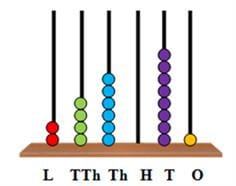# Test: Introduction to Numbers- 1

## 10 Questions MCQ Test Mathematics for Class 4 | Test: Introduction to Numbers- 1

Description
Attempt Test: Introduction to Numbers- 1 | 10 questions in 15 minutes | Mock test for Class 4 preparation | Free important questions MCQ to study Mathematics for Class 4 for Class 4 Exam | Download free PDF with solutions
QUESTION: 1

### Which of the following is true for the number 82,951?

Solution:

The statement ‘2 is the face value of 2 in 82,951’ is true.
The false statements can be corrected as 8 is the face value of 8 in 82,951.
900 is the place value of 9 in 82,951.
50 is the place value of 5 in 82,951.

QUESTION: 2

### Which of the following is the smallest 5-digit number?

Solution:

The number 10,000 is the smallest 5-digit number.
It comes just after the greatest 4-digit number 9999.

QUESTION: 3

### What is the place value of the underlined digit in the number 7,65,730?

Solution:

The place value of a number is the value of the digit in the given number.
The place value of the underlined digit in the number 7,65,730 is 7,00,000 as it is in the lakhs place.

QUESTION: 4

Which of the following shows the first three places from the right in a 5-digit number?

Solution:

The ones period includes the ones, tens and hundreds place which are three places from the right of a 5-digit number.
Hence, the ones period is the right answer.

QUESTION: 5

Which of the following can also be written as 4,50,000?

Solution:

There are 45 ten thousands in 4,50,000.
Hence, it can also be written as forty-five ten thousands.

QUESTION: 6

One lakh can be expressed as ____________.

Solution:

1,00,000 is equal to 100 thousands.
1,00,000 is read as one lakh.
Hence, 1,00,000 can be expressed as 100 thousands.

QUESTION: 7

Which of the following options is read as thirty-five thousand six hundred and thirty-five?

Solution:

There are three ten thousands, five thousands, six hundreds, three tens and five ones in the number 35,635. Hence, we read 35,635 as thirty-five thousand six hundred and thirty-five.

QUESTION: 8

Which of the following is the standard form for the given expanded form? 40,000 + 6,000 + 700 + 80 + 1

Solution:

In the standard form, we write 40,000 + 6,000 + 700 + 80 + 1 as 46,781.

QUESTION: 9

Which of the following is one more than 99,999?

Solution:

99,999 is the greatest 5-digit number.
When 1 is added to 99,999, we get the 6-digit number 1,00,000.

QUESTION: 10

Which of the following is the number which can be represented using the given abacus?Solution:

There are 2 beads in the lakhs place, 4 beads in the ten thousands place, 6 beads in the thousands place, 0 beads in the hundreds place, eight beads in the tens place and one bead in the ones place.
Hence, the number on the abacus is 2,46,081.Use Code STAYHOME200 and get INR 200 additional OFF Use Coupon Code